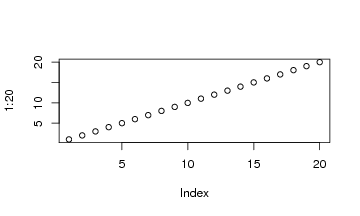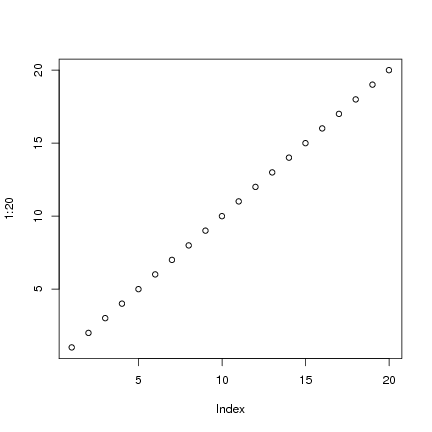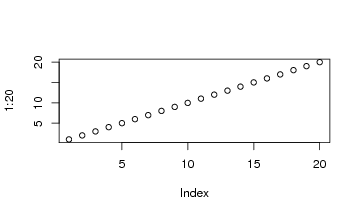DATA MINING
Desktop Survival Guide
by Graham Williams### Figure Sizes

Figures will be typeset within the document, when the option fig=TRUE is set. The default is to typeset the figure to be 80% the width of the text width. This can be reset with something like `\setkeys{Gin}{width=0.75\textwidth}`.

By default the figures generated by Sweave (as .eps and .png files) are 7x7 inches. Changing, for example, the default output size with the pdf function does not affect the pdf produced by Sweave. The following example illustrates:

 ```> pdf.options(width=5, height=3) > pdf("reporting_pdf_size.pdf") > plot(1:20) > dev.off() ```

 ```pdf 2 ```However, within Sweave:

 ```> pdf.options(width=5, height=3) > plot(1:20) ```Instead, the options are set using the width and height options within the Sweave header for that block:

 ```> plot(1:20) ```These dimensions can be set for the following portion of the document with `\Swea``veOpts{width=5, height=3}`.